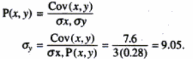Courses

If the coefficient of correlation between x and y is 0.28, covariance between x and y is 7.6, and the variance of x is 9, then the standard deviation of the y series isa)9.8b)10.1c)9.05d)10.05Correct answer is option 'C'. Can you explain this answer?

Related TestKajal Rathore Dec 06, 2018
N the given problem it is SD of x is 3: (or Variance of x is 9). As Variance = (Sx)^2. We know the relation : correlation coefficient (r) = Cov (x,y) / (Sx * Sy) so, 0.28 = 7.6 / (3 * Sy) From here we get the value of SD of Y : Sy = 9.05.SOURAV KUMAR Jan 07, 2020N the given problem it is SD of x is 3: (or Variance of x is 9). As Variance = (Sx)^2. We know the relation : correlation coefficient (r) = Cov (x,y) / (Sx * Sy) so, 0.28 = 7.6 / (3 * Sy) From here we get the value of SD of Y : Sy = 9.05.

Top Courses for JEERelated ContentPPT: Standard Deviation & Coefficient of VarianceDoc | 9 PagesCovariance and CorrelationDoc | 1 Page